6.2.1 Formats

6.2.1.1 General

RLC PDU is a bit string. In the figures in sub clause 6.2.1.2 to 6.2.1.6, bit strings are represented by tables in which the first and most significant bit is the left most bit of the first line of the table, the last and least significant bit is the rightmost bit of the last line of the table, and more generally the bit string is to be read from left to right and then in the reading order of the lines.

RLC SDUs are bit strings that are byte aligned (i.e. multiple of 8 bits) in length. An RLC SDU is included into an RLC PDU from first bit onward.

6.2.1.2 TMD PDU

TMD PDU consists only of a Data field and does not consist of any RLC headers.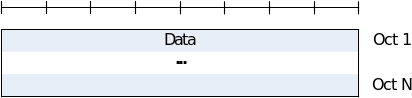Figure 6.2.1.2-1: TMD PDU

6.2.1.3 UMD PDU

UMD PDU consists of a Data field and an UMD PDU header.

UMD PDU header consists of a fixed part (fields that are present for every UMD PDU) and an extension part (fields that are present for an UMD PDU when necessary). The fixed part of the UMD PDU header itself is byte aligned and consists of a FI, an E and a SN. The extension part of the UMD PDU header itself is byte aligned and consists of E(s) and LI(s).

Except for NB-IoT, an UM RLC entity is configured by RRC to use either a 5 bit SN or a 10 bit SN. For NB-IoT, an UM RLC entity uses a 5 bit SN. When the 5 bit SN is used, the length of the fixed part of the UMD PDU header is one byte. When the 10 bit SN is used, the fixed part of the UMD PDU header is identical to the fixed part of the AMD PDU header, except for D/C, RF and P fields all being replaced with R1 fields. The extension part of the UMD PDU header is identical to the extension part of the AMD PDU header (regardless of the configured SN size).

An UMD PDU header consists of an extension part only when more than one Data field elements are present in the UMD PDU, in which case an E and a LI are present for every Data field element except the last. Furthermore, when an UMD PDU header consists of an odd number of LI(s), four padding bits follow after the last LI.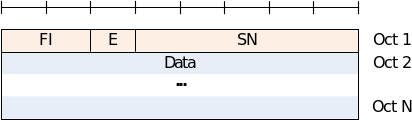Figure 6.2.1.3-1: UMD PDU with 5 bit SN (No LI)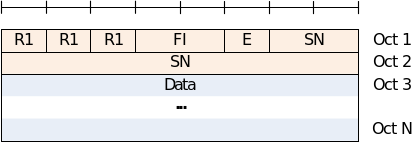Figure 6.2.1.3-2: UMD PDU with 10 bit SN (No LI)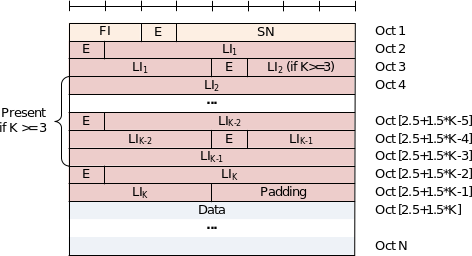Figure 6.2.1.3-3: UMD PDU with 5 bit SN (Odd number of LIs, i.e. K = 1, 3, 5, …)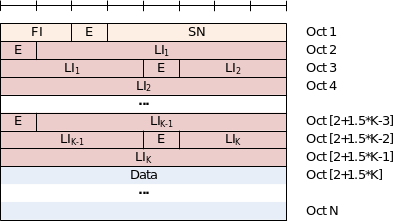Figure 6.2.1.3-4: UMD PDU with 5 bit SN (Even number of LIs, i.e. K = 2, 4, 6, …)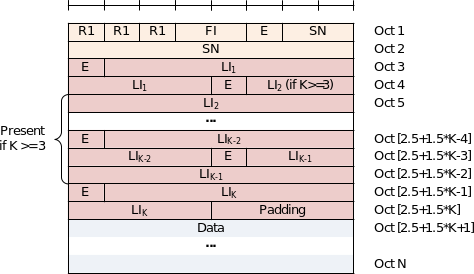Figure 6.2.1.3-5: UMD PDU with 10 bit SN (Odd number of LIs, i.e. K = 1, 3, 5, …)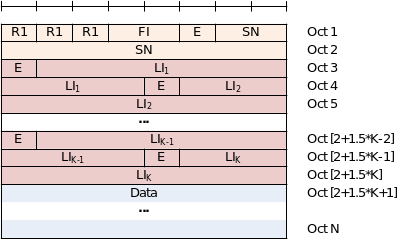Figure 6.2.1.3-6: UMD PDU with 10 bit SN (Even number of LIs, i.e. K = 2, 4, 6, …)

6.2.1.4 AMD PDU

AMD PDU consists of a Data field and an AMD PDU header.

AMD PDU header consists of a fixed part (fields that are present for every AMD PDU) and an extension part (fields that are present for an AMD PDU when necessary). The fixed part of the AMD PDU header itself is byte aligned and consists of a D/C, a RF, a P, a FI, an E and a SN. The extension part of the AMD PDU header itself is byte aligned and consists of E(s) and LI(s).

The transmitting side and the receiving side of an AM RLC entity are configured by RRC to use either a 10 bit SN or a 16 bit SN. The length of the fixed part of the AMD PDU header is two and three bytes respectively. The default values for SN field length used by an AM RLC entity is 10 bits.

An AMD PDU header consists of an extension part only when more than one Data field elements are present in the AMD PDU, in which case an E and a LI are present for every Data field element except the last. Furthermore, when an AMD PDU header consists of an odd number of LI(s) and the length of the LI field is 11 bits, four padding bits follow after the last LI. The default value for LI field length used by an AM RLC entity is 11 bits.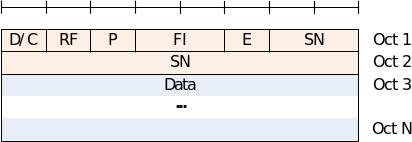Figure 6.2.1.4-1: AMD PDU with 10 bit SN (length of LI field is 11 bits) (No LI)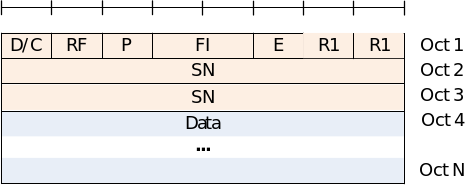Figure 6.2.1.4-1a: AMD PDU with 16 bit SN (length of LI field is 11 bits) (No LI)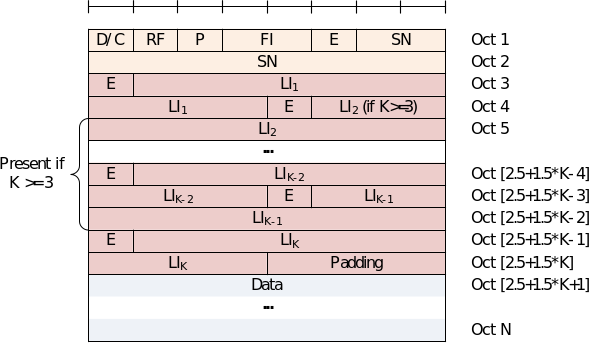Figure 6.2.1.4-2: AMD PDU with 10 bit SN (length of LI field is 11 bits) (Odd number of LIs, i.e. K = 1, 3, 5, …)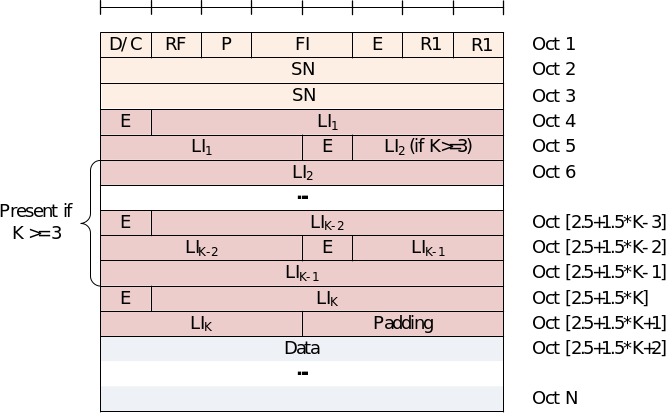Figure 6.2.1.4-2a: AMD PDU with 16 bit SN (length of LI field is 11 bits) (Odd number of LIs, i.e. K = 1, 3, 5, …)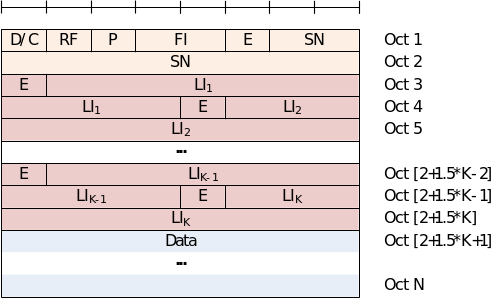Figure 6.2.1.4-3: AMD PDU with 10 bit SN (length of LI field is 11 bits) (Even number of LIs, i.e. K = 2, 4, 6, …)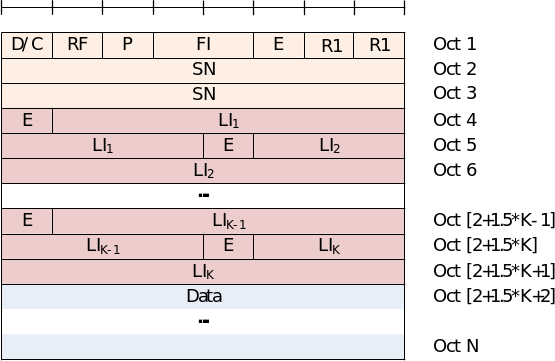Figure 6.2.1.4-3a: AMD PDU with 16 bit SN (length of LI field is 11 bits) (Even number of LIs, i.e. K = 2, 4, 6, …)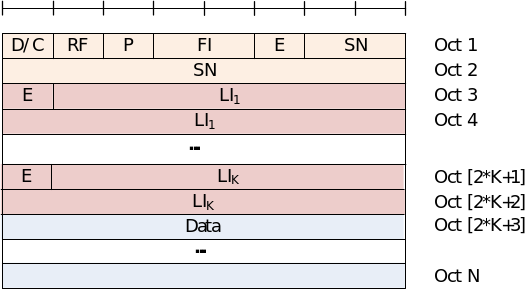Figure 6.2.1.4-4: AMD PDU with 10 bit SN (length of LI field is 15 bits)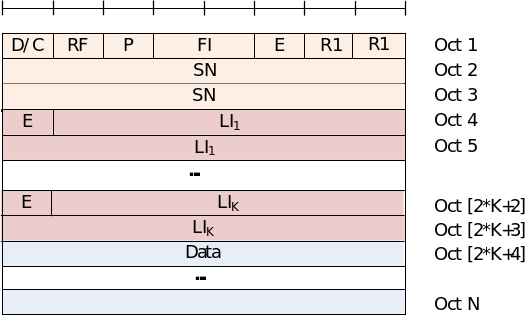Figure 6.2.1.4-4a: AMD PDU with 16 bit SN (length of LI field is 15 bits)

6.2.1.5 AMD PDU segment

AMD PDU segment consists of a Data field and an AMD PDU segment header.

AMD PDU segment header consists of a fixed part (fields that are present for every AMD PDU segment) and an extension part (fields that are present for an AMD PDU segment when necessary). The fixed part of the AMD PDU segment header itself is byte aligned and consists of a D/C, a RF, a P, a FI, an E, a SN, a LSF and a SO. The extension part of the AMD PDU segment header itself is byte aligned and consists of E(s) and LI(s).

The transmitting side and the receiving side of an AM RLC entity are configured by RRC to use either a 10 bit SN or a 16 bit SN. When a 10 bit SN is used, the SO field is 15 bits, and when a 16 bit SN is used, the SO field is 16 bits. The length of the fixed part of the AMD PDU segment header is four and five bytes respectively. The default values for SN field length and SO field length used by an AM RLC entity are 10 bits and 15 bits, respectively.

An AMD PDU segment header consists of an extension part only when more than one Data field elements are present in the AMD PDU segment, in which case an E and a LI are present for every Data field element except the last. Furthermore, when an AMD PDU segment header consists of an odd number of LI(s) and the length of the LI field is 11 bits, four padding bits follow after the last LI. The default value for LI field length used by an AM RLC entity is 11 bits.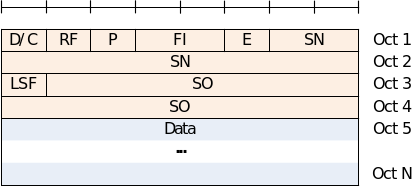Figure 6.2.1.5-1: AMD PDU segment with 10 bit SN (No LI)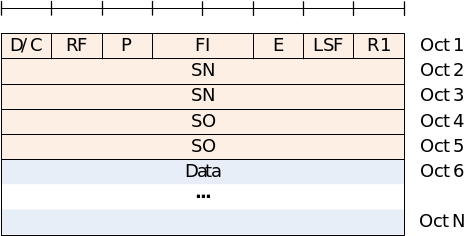Figure 6.2.1.5-1a: AMD PDU segment with 16 bit SN and with 16 bit SO (No LI)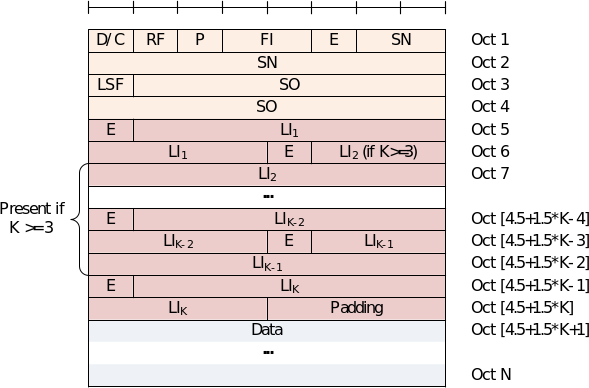Figure 6.2.1.5-2: AMD PDU segment with 10 bit SN (length of LI field is 11 bits) (Odd number of LIs, i.e. K = 1, 3, 5, …)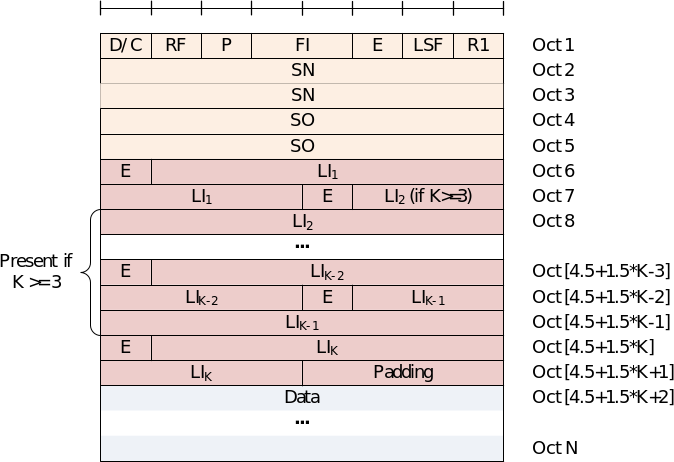Figure 6.2.1.5-2a: AMD PDU segment with 16 bit SN and with 16 bit SO (length of LI field is 11 bits) (Odd number of LIs, i.e. K = 1, 3, 5, …)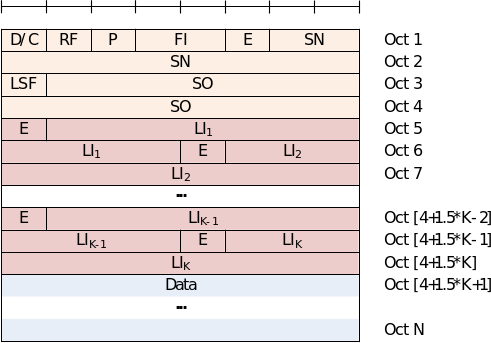Figure 6.2.1.5-3: AMD PDU segment with 10 bit SN (length of LI field is 11 bits) (Even number of LIs, i.e. K = 2, 4, 6, …)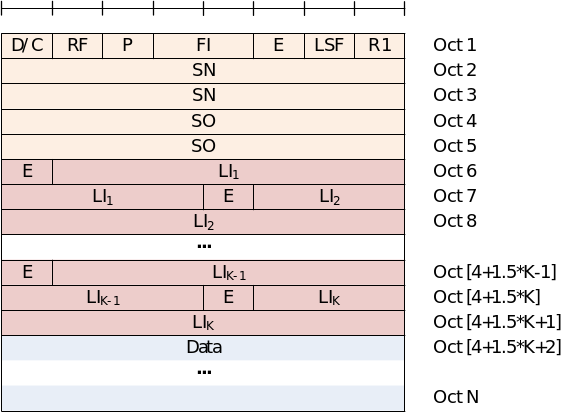Figure 6.2.1.5-3a: AMD PDU segment with 16 bit SN and with 16 bit SO (length of LI field is 11 bits) (Even number of LIs, i.e. K = 2, 4, 6, …)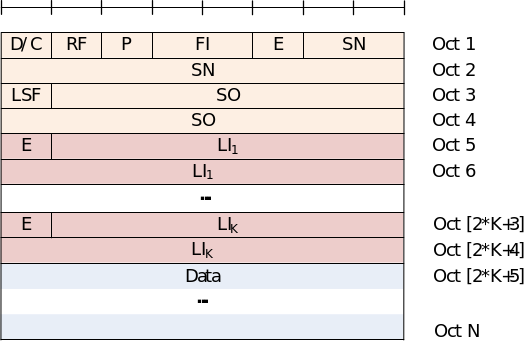Figure 6.2.1.5-4: AMD PDU segment with 10 bit SN (length of LI field is 15 bits)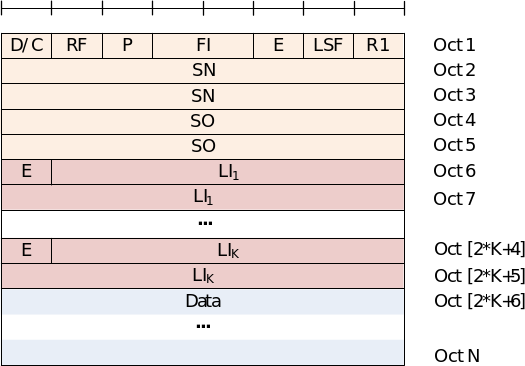Figure 6.2.1.5-4a: AMD PDU segment with 16 bit SN and with 16 bit SO (length of LI field is 15 bits)

6.2.1.6 STATUS PDU

STATUS PDU consists of a STATUS PDU payload and a RLC control PDU header.

RLC control PDU header consists of a D/C and a CPT field.

The STATUS PDU payload starts from the first bit following the RLC control PDU header, and it consists of one ACK_SN and one E1, zero or more sets of a NACK_SN, an E1 and an E2, and possibly a set of a SOstart and a SOend for each NACK_SN. When necessary one to seven padding bits are included in the end of the STATUS PDU to achieve octet alignment.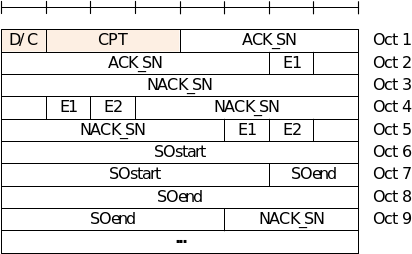Figure 6.2.1.6-1: STATUS PDU with 10 bit SN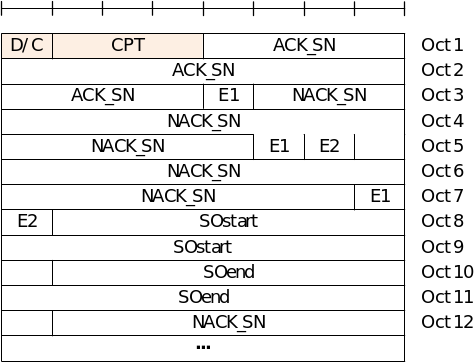Figure 6.2.1.6-2: STATUS PDU with 16 bit SN and with 16 bit SOstart and SOend fields